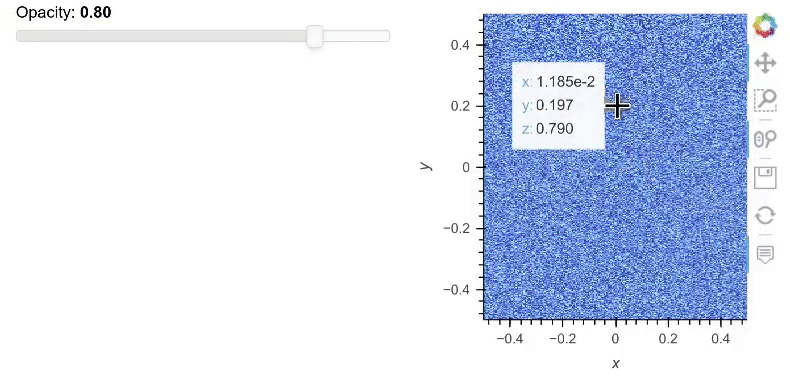# Change opacity while keeping the extent of wheel zoom

If we plot an image, zoom in, and then want to change the opacity using panel. It would return back to the default extent. It’s more convenient to only change the opacity, especially for large data.

Here’s the example:

``````import numpy as np
import holoviews as hv
from bokeh.models import HoverTool
import panel as pn
hv.extension("bokeh")

tooltips = [
('x', '\$x'),
('y', '\$y'),
('z', '@image'),
]

hover = HoverTool(tooltips=tooltips)
pn_opacity = pn.widgets.FloatSlider(name='Opacity', value=0.8, start=0, end=1, step=0.1)

@pn.depends(pn_opacity_value = pn_opacity.param.value)
def test(pn_opacity_value):
return hv.Image(np.random.rand(2000,200)).opts(tools=[hover], active_tools=['wheel_zoom'], alpha=pn_opacity_value)

DashboardPanel = pn.Row(pn.Column(pn.Column(pn_opacity)), test)
DashboardPanel.servable()
``````Replace `test` by `hv.DynamicMap(test)` in your dashboard

2 Likes

Thanks. If the image is rasterized, then `Dynamicmap` won’t work. Do you have any idea?

### Code:

``````import numpy as np
import holoviews as hv
from bokeh.models import HoverTool
import panel as pn
hv.extension("bokeh")

tooltips = [
('x', '\$x'),
('y', '\$y'),
('z', '@image'),
]

hover = HoverTool(tooltips=tooltips)
pn_opacity = pn.widgets.FloatSlider(name='Opacity', value=0.8, start=0, end=1, step=0.1)

@pn.depends(pn_opacity_value = pn_opacity.param.value)
def test(pn_opacity_value):
return rasterize(hv.Image(np.random.rand(2000,200)))\
.opts(tools=[hover], active_tools=['wheel_zoom'], alpha=pn_opacity_value)

DashboardPanel = pn.Row(pn.Column(pn.Column(pn_opacity)), hv.DynamicMap(test))
DashboardPanel.servable()
``````

### Error:

``````~/miniconda3/envs/knmi_arctic/lib/python3.8/site-packages/holoviews/plotting/util.py in collate(obj)
61         if obj.type in [DynamicMap, HoloMap]:
62             obj_name = obj.type.__name__
---> 63             raise Exception("Nesting a %s inside a DynamicMap is not "
64                             "supported. Ensure that the DynamicMap callback "
65                             "returns an Element or (Nd)Overlay. If you have "

Exception: Nesting a DynamicMap inside a DynamicMap is not supported. Ensure that the DynamicMap callback returns an Element or (Nd)Overlay. If you have applied an operation ensure it is not dynamic by setting dynamic=False.
``````

so in your case you can do this:

``````img = rasterize(hv.Image(np.random.rand(2000,200)).opts(tools=[hover], active_tools=['wheel_zoom'], alpha=pn_opacity.value))
DashboardPanel = pn.Row(pn.Column(pn.Column(pn_opacity)), img.apply.opts(alpha=pn_opacity.param.value))
DashboardPanel.servable()
``````
1 Like

Ha, appreciate a lot! It works well.

From where would a user be able to know that `img.apply.opts(alpha=pn_opacity.param.value)` is needed? how did you know?

https://holoviews.org/user_guide/Responding_to_Events.html

section Using `.apply.opts`

1 Like